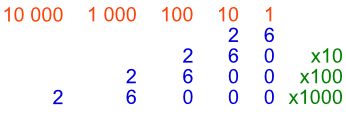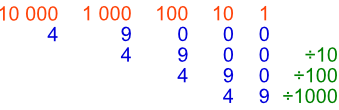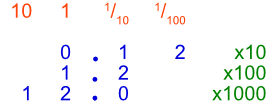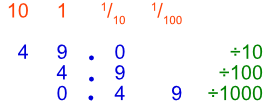Multiplying and Dividing in 10s

# Multiplying and Dividing in 10s

Basic

Multiplying by 10, 100, 1000, moves the digits in each column (units, 10s, 100s, etc) to the left:

• multiplying by 10 moves all the digits 1 column to the left;

• multiplying by 100 moves the digits 2 columns to the left;

• multiplying by 1000 moves the digits 3 columns to the left.Dividing by 10, 100, 1000 moves the digits in each column to the right:

• dividing by 10 moves them 1 digit to the right;

• dividing by 100 is 2 digits right;

• dividing by 1000 is 3 digits right.The same is true for decimal numbers. Although the digits move, the decimal point stays in the same place.## Examples

1. What is 34 x 100?